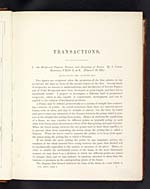# James Clerk Maxwell (1831-1879)

## On reciprocal figures, frames and diagrams of forces

TRANSACTIONS.

I.—On Reciprocal Figures, Frames, and Diagrams of Forces. By J. CLERK
MAXWELL, F.R.SS. L. & E. (Plates I. II. III.)

Two figures are reciprocal when the properties of the first relative to the
second are the same as those of the second relative to the first. Several kinds
of reciprocity are known to mathematicians, and the theories of Inverse Figures
and of Polar Reciprocals have been developed at great length, and have led to
remarkable results. I propose to investigate a different kind of geometrical
reciprocity, which is also capable of considerable development, and can be
applied to the solution of mechanical problems.

A Frame may be defined geometrically as a system of straight lines connect-
ing a number of points. In actual structures these lines are material pieces,
beams, rods, or wires, and may be straight or curved; but the force by which
each piece resists any alteration of the distance between the points which it joins
acts in the straight line joining those points. Hence, in studying the equilibrium
of a frame, we may consider its different points as mutually acting on each
other with forces whose directions are those of the lines joining each pair of points.
When the forces acting between the two points tend to draw them together, or
to prevent them from separating, the action along the joining line is called a
Tension. When the forces tend to separate the points, or to keep them apart,
the action along the joining line is called a Pressure.

If we divide the piece joining the points by any imaginary section, the
resultant of the whole internal force acting between the parts thus divided will
be mechanically equivalent to the tension or pressure of the piece. Hence, in
order to exhibit the mechanical action of the frame in the most elementary
manner, we may draw it as a skeleton, in which the different points are joined
by straight lines, and we may indicate by numbers attached to these lines the
tensions or pressures in the corresponding pieces of the frame.

The diagram thus formed indicates the state of the frame in a way which is

VOL. XXVI. PART I.

AView larger image